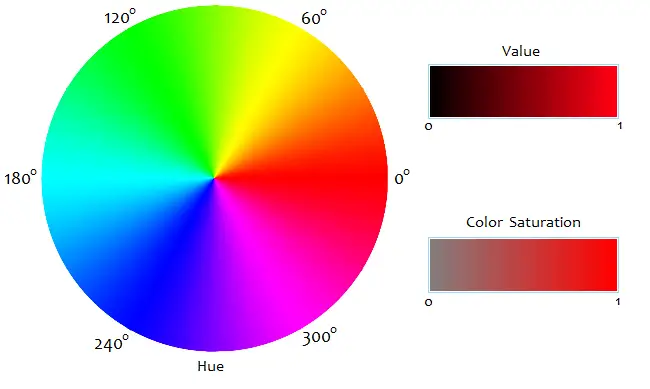# RGB to HSV, HSV to RGB Conversion Calculator

RGB/HSV Conversion Calculator
RGB to HSV

HSV to RGB

 ColorColor
R, G, and B range from 0 to 255. Hue ranges from 0° to 360°. Saturation ranges from 0 to 1. Value ranges from 0 to 1.

The HSV color model describes colors according to their Hue, Saturation, and Value. In some computer graphics programs, it is used as an alternative to the RGB system to quantify colors.

In HSV, hue is a number in the interval [0, 360). A color's hue is its general position on a color wheel, where red is at 0°, green is at 120°, and blue is at 240°. For example the RGB code of a yellow/orange color has high red and green components and a low blue component, with the red level slightly higher than the green. On the color wheel, the angle of this hue is a little less than 60°. The hue of any neutral color--white, gray, or black--is set at 0°.

Value, in HSV, is the highest value among the three R, G, and B numbers. This number is divided by 255 to scale it between 0 and 1. In terms of perception, HSV Value represents how light, bright, or intense a color is. Value does not distinguish white and pure colors, all of which have V = 1.

HSV Saturation measures how close a color is to the grayscale. S ranges from 0 to 1. White, gray, and black all have a saturation level of 0. Brighter, purer colors have a saturation near 1. In other color models that include a saturation component, the precise mathematical definition of S may vary. See HSI and HSL.### Converting RGB to HSV

Given three numbers R, G, and B (each between 0 and 255), you can first define m and M with the relations

M = max{R, G, B}
m = min{R, G, B}.

And then V and S are defined by the equations

V = M/255
S = 1 - m/M     if M > 0
S = 0              if M = 0.

As in the HSI and HSL color schemes, the hue H is defined by the equations

H = cos-1[ (R - ½G - ½B)/√R² + G² + B² - RG - RB - GB ]            if G ≥ B, or
H = 360 - cos-1[ (R - ½G - ½B)/√R² + G² + B² - RG - RB - GB ]    if B > G.

Inverse cosine is calculated in degrees.

### Converting HSV to RGB

Given the values of H, S, and V, you can first compute m and M with the equations

M = 255V
m = M(1-S).

Now compute another number, z, defined by the equation

z = (M-m)[1 - |(H/60)mod_2 - 1|],

where mod_2 means division modulo 2. For example, if H = 135, then (H/60)mod_2 = (2.25)mod_2 = 0.25. In modulo 2 division, the output is the remainder of the quantity when you divide it by 2.

Now you can compute R, G, and B according to the angle measure of H. There are six cases. When 0 ≤ H < 60,

R = M
G = z + m
B = m.

If 60 ≤ H < 120,

R = z + m
G = M
B = m.

If 120 ≤ H < 180,

R = m
G = M
B = z + m.

When 180 ≤ H < 240,

R = m
G = z + m
B = M.

When 240 ≤ H < 300,

R = z + m
G = m
B = M.

And if 300 ≤ H < 360,

R = M
G = m
B = z + m.